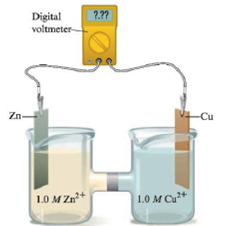# Consider the following galvanic cell: a. Label the reducing agent and the oxidizing agent, and describe the direction of the electron flow. b. Determine the standard cell potential. c. Which electrode increases in mass as the reaction proceeds, and which electrode decreases in mass?### Chemistry: An Atoms First Approach

2nd Edition
Steven S. Zumdahl + 1 other
Publisher: Cengage Learning
ISBN: 9781305079243

#### Solutions

Chapter
Section### Chemistry: An Atoms First Approach

2nd Edition
Steven S. Zumdahl + 1 other
Publisher: Cengage Learning
ISBN: 9781305079243
Chapter 17, Problem 36E
Textbook Problem
1 views

## Consider the following galvanic cell:a. Label the reducing agent and the oxidizing agent, and describe the direction of the electron flow.b. Determine the standard cell potential.c. Which electrode increases in mass as the reaction proceeds, and which electrode decreases in mass?

(a)

Interpretation Introduction

Interpretation:

The set up of galvanic cell is given. Various questions based on the given galvanic cell are to be answered.

Concept introduction:

The galvanic cell consists of two half-cell. On half-cell consist of anode and the other half-cell consists of cathode. The oxidation occurs over the anode while the reduction occurs over the cathode. So, the species at anode acts as a reducing agent while the species at the cathode acts as an oxidizing agent.

### Explanation of Solution

The zinc electrode acts as the reducing agent while the copper electrode acts as the oxidizing agent and the flow of electrons occur from zinc to copper electrode.

The labeled diagram of galvanic cell is represented as,

Figure 1

The reactions that take place in cell are as follows,

ZnZn2++2e-ox=0

(b)

Interpretation Introduction

Interpretation:

The set up of galvanic cell is given. Various questions based on the given galvanic cell are to be answered.

Concept introduction:

The galvanic cell consists of two half-cell. On half-cell consist of anode and the other half-cell consists of cathode. The oxidation occurs over the anode while the reduction occurs over the cathode. So, the species at anode acts as a reducing agent while the species at the cathode acts as an oxidizing agent.

(c)

Interpretation Introduction

Interpretation:

The set up of galvanic cell is given. Various questions based on the given galvanic cell are to be answered.

Concept introduction:

The galvanic cell consists of two half-cell. On half-cell consist of anode and the other half-cell consists of cathode. The oxidation occurs over the anode while the reduction occurs over the cathode. So, the species at anode acts as a reducing agent while the species at the cathode acts as an oxidizing agent.

### Still sussing out bartleby?

Check out a sample textbook solution.

See a sample solution

#### The Solution to Your Study Problems

Bartleby provides explanations to thousands of textbook problems written by our experts, many with advanced degrees!

Get Started

Find more solutions based on key concepts
Fruit punch sweetened with grape juice concentrate can contain as much sugar as fruit punch sweetened with high...

Nutrition: Concepts and Controversies - Standalone book (MindTap Course List)

What are sister chromatids?

Human Heredity: Principles and Issues (MindTap Course List)

If the velocity of a particle is nonzero, can the particles acceleration be zero? Explain.

Physics for Scientists and Engineers, Technology Update (No access codes included)

How many cubic centimeters (cm3) are in one cubic meter (m3)?

Physics for Scientists and Engineers: Foundations and Connections

What factors affect the depth of the photic zone? Could there be a photocline in the ocean?

Oceanography: An Invitation To Marine Science, Loose-leaf Versin